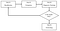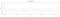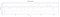# Univariate Modelling for Financial Data using R

This post will guide you through several statistical techniques to analyse time-series data with special applications to finance. We will be using R for the analysis.Photo by Chris Liverani on Unsplash

The main objective of our analysis is to model the stock prices of Facebook, Inc. When it comes to analysing financial data, we can use mainly two types of models, namely,

1. Univariate Models

In univariate models, we make the value predictions using only information contained in our own past values or current and past values of an error term. However, in multivariate models, we study the relationships between several variables. In this analysis, we will be focusing on univariate models for predicting stock prices.

## Univariate Models

Univariate models are usually a-theoretical, which means they are not based on theory and are constructed to capture important features of the data itself. These types of models are mainly useful when the factors influencing the variables of interest are not observable or are measured at a lower frequency. For example, let’s say we want to predict the daily stock returns but possible explanatory variables such as macroeconomic conditions are only available quarterly. So in such cases, we can use univariate models.

The most common class of univariate time series model is the ARIMA (Autoregressive Integrated Moving Average)) model. We will now see how to develop an ARIMA model for predicting stock prices using R.

# Dataset

The selected dataset consists of 5years of daily stock prices of Facebook, Inc (FB) from 01st of January 2015 to the present. However, there are only 1517 observations in the data as the data is not available for several days due to some reasons such as holidays.

You can download the data from here.

## Variables

1. Open: the price at which financial security opens in the market when trading begins.

2. Close: the last price at which a stock trades during a regular trading session.

3. Adjusted close: amended closing price of a stock to reflect its value after accounting for any corporate actions.

4. High: the highest intraday price of a stock in the most recent (or current) trading session.

5. Low: the lowest intraday price of a stock in the most recent (or current) trading session.

6. Volume: the number of shares of a security traded during the day.

For the univariate analysis, the variable Adjusted close is selected as it is often used when examining the historical returns or doing a detailed analysis of past performance.

The first step is to load the required libraries.

`#import  librarieslibrary(“zoo”)library(“strucchange”)library(“fpp2”)library(“tseries”)library(“hydroTSM”)library(“xts”)library(“MuMIn”)library(“aTSA”)library(“fGarch”)library(“rugarch”)library(“forecast”)`

Before modelling the data, let’s transform the raw data into log values, which is a commonly used method in Financial Econometrics to stabilize the variance of data.

`close=FB[,"Adj.Close"]close_log = log(close)#log transformationclose_vals <- ts(data = close_log, frequency = 356, start = c(2015,1))`

# Let’s start modelling…

The first step of the analysis is to identify which model to apply to our time series data. Box and Jenkins (1976) came up with a methodology to answer this question. The methodology has several steps:

1.Identification — values of p,d,q

2.Estimation — using OLS or MLE

3.Diagnostic checking — assessing model fit

4.ForecastingBox-Jenkins methodology

This is an iterative process. For instance, if step 3 indicates that the model is not good enough, go back to step 1.

## Some preprocessing…

Now, we will try to plot how our target variable, the Adjusted close price has changed over time using a time series plot.

`plot(close_vals, col = “Blue4”)`

So, looking at the above graph, we can observe that the data is non-stationary. However, before checking for the stationarity in data, it is more appropriate to check if there are any structural breaks and seasonal effects within the data, as it would affect the results of the stationarity tests.

`#Check for structural breaksclose_brk <- breakpoints(close_vals~1, h=0.1)summary(close_brk)plot(close_brk)#plottingbreakdates(close_brk, breaks =7)plot(close_vals)lines(fitted(close_brk, breaks=7), col=4)lines(confint(close_brk, breaks=7))`

When checking for the structural breaks within the data, R suggests 7 breaks as the optimal number of breaks to be included.

To check the effects of the structural breaks, let’s fit a regression including dummy variables for each of the structural breaks.

`FB\$break1 <- 1FB\$break1[1:257] <- 0FB\$break2 <- 1FB\$break2[1:482] <- 0FB\$break3 <- 1FB\$break3[1:787] <- 0FB\$break4 <- 1FB\$break4[1:1007] <- 0FB\$break5 <- 1FB\$break5[1:1338] <- 0FB\$break6 <- 1FB\$break6[1:1558] <- 0FB\$break7 <- 1FB\$break7[1:1965] <- 0FB\$close_log <- close_loglm1<-lm(close_log~break1+break2+break3+break4+break5+break6+break7,data = FB)summary(lm1)`

According to the summary results of the fitted model, all the terms are highly statistically significant. This means all seven structural breaks have significant impacts on stock prices.

When investigating the residuals of the above model, it can also be concluded that the model has stationary residuals.

`resids1 <- lm1\$residualsadf.test(resids1)`

However, if we consider all 7 structural breaks within the data, it will cause some practical problems when doing the analysis. For instance, splitting the dataset in each break would result in a very small dataset that cannot capture any seasonal effects. Therefore, we will split the dataset into two based on the most significant structural break out of all seven breaks reported by R.

`plot(close_vals)lines(fitted(close_brk, breaks=1), col=4)lines(confint(close_brk, breaks=1))`
`#Create the  new dataset by  splittingclose_vals_latest <-close_vals[798:2202]close_vals_latest_ts <- ts(data = close_vals_latest, frequency = 356, start = c(2017,86))`

After splitting, the new dataset contains 1405 data points from March 27, 2017, to January 11, 2021. Let’s have a look at the time series plot of the new dataset.

`#plottingplot(close_vals_latest_ts, col = “Blue4”, ylab = “Adjusted close price”)`

Now, we have controlled for the structural breaks within the data. The next step is to check whether there are any seasonal effects within the dataset. Let’s plot the average stock price for each month to see if there is any monthly seasonal pattern.

`#Check for monthly seasonal effectsdate = seq(as.Date(‘2017–03–9’), as.Date(‘2021–01–11’), by = ‘days’)means<- monthlyfunction(data.matrix(close_vals_latest_ts), mean, na.rm=TRUE, dates = date,out.type = ‘data.frame’)M_means <- c(means,means,means,means,means,means,means,means,means,means,means,means)plot(M_means, ylim = c(4,6), type = “b”, col = “Red”,xaxt = ’n’, ylab=’Monthly averages’,xlab = ‘Month’) #plot monthly averagesaxis(1, at=1:12, labels=c(“March”,”April”,”May”,”June”,”July”,”August”,”September”,”October”,”November”,”December”,”January”,”February”), cex.axis=1)`

As shown in the following figure, there cannot be seen any monthly seasonal pattern in the data. We can explore this further, using the plots of auto-correlation function (ACF) and partial autocorrelation function (PACF), when deciding the number of autoregressive terms and moving average terms to be included in the ARIMA model.

## Identification

In the identification stage, the primary task is to determine the number of auto-regressive terms (p), the order of integration (d) and the number of moving average terms (q). The new dataset after control for the structural breaks also seems to be non-stationary, as we’ve seen previously. Therefore, it is better for us to do a formal test to see the stationarity of the data. We can use an Augmented Dickey-Fuller (ADF) test for this purpose.

• H0: Process is non-stationary.
`#Box-Jenkins methology for estimating ARIMA model##Identification###initial guess for dadf.test(close_vals_latest_ts) #Check for stationarity`

The p-value of the test is 0.4191, which is lower than 0.05. That means we do not reject the null. Hence, at a 5% significance level, there is evidence to say that the process is non-stationary.

So, let’s difference the data by one lag to make it stationary.

`d.close_vals=diff(close_vals_latest_ts,differences = 1) #Differeningadf.test(d.close_vals) #check for stationarity of the difference dataplot.ts(d.close_vals, col = “bLUE4”, ylab = “Close price returns (differenced close price)”)`

The test results of the ADF test and the plot of the differenced data are exhibited below.

Now, we can see that the process has become stationary after differencing. That means, we can conclude that the process is I(1), which means, d=1. Next, we will find the values of p and q using the ACF and PACF plots.

`###Initial guesses for p and qacf(d.close_vals, lag=30, ylim = c(-0.1,0.2))pacf(d.close_vals, lag=30)`ACF plot for the dataPACF plot for the data

In both ACF and PACF only the first lag has reported a significant spike, which means both the values of p and q are 1. Therefore, we will estimate an ARIMA (1, 1, 1) model for the data. In addition, we can also observe that there are no seasonal effects in the ACF and PACF plots as well.

## Estimation

Now, let’s fit the selected model and see the results.

`##Estimationarima_1 <- Arima(close_vals_latest_ts, order=c(1,1,1))arima_1`

In order to confirm that the selected model is appropriate, let’s fit another model using the ‘autoarima’ function provided by R.

`fit <- auto.arima(close_vals_latest_ts, seasonal=FALSE) #auto.arima function to ensure the model selectionfit`

As you can see, the values of d and q are still the same as in the previous model, yet R suggests that the value of p should be zero. Now, we need to select the most appropriate model for the data out of these two models. There are several information criteria we can use for this task.

1. Akaike’s information criterion (AIC)

3. Schwarz’s Bayesian Information Criterion (SBIC or simply BIC)

4. Hannan-Quinn Criterion (HQIC)

Let's use AIC, AICc and BIC as our criteria in this case for selecting the best model.

`###Information criterion AIC(arima_1,fit)BIC(arima_1,fit)AICc(arima_1,fit)`

Out of the 3 information criteria, both the AIC and AICc suggest that the ARIMA (1, 1, 1) model is the most appropriate for predicting the stock prices. Therefore, we can select the model, ARIMA (1, 1, 1) as the final model for the analysis.

## Diagnostic Checking

As previously mentioned, at this stage we are trying to assess our model fit, which means, to check whether the residuals of the estimated model are white noise. We can use two methods for this, namely, the Ljung-Box test and plots of the residuals. Let’s first see what the results of the Ljung-Box (LB) test say.

`##Diagnostic checkingcheckresiduals(arima_1)`
• H0: Residuals are white noise.

The p-value of the test is 0.3999, which is greater than 0.05. That means, we do not reject H0. Therefore, we can say that the error term of the model, ARIMA (1,1,1) is white noise at a 95% confidence level. Now, let’s also have a look at the plots of the residuals of the model.

Looking at the histogram of the residuals, we can conclude that the residuals are normally distributed. The time-series plot of the residuals also suggests that the process is stationary. Though few lags within the ACF plot have significant spikes, that is insignificant compared to the total number of lags displayed in the plot. Thus, the overall conclusion that can be derived from the plots of the residuals is the same as the LB test, which is that the residuals are white noise.

Now, let’s move to the last part of our analysis, which is to make the forecast.

## Forecasting

First, we need to split the data into a train set and a test set. We will split it in the ratio of 7:3. Then, the training data set which is used for training the model consists of 991 observations. The test is used for validating the model performance and, it contains 414 data points. Now, let’s train data using the above two models, and then make some forecasts for the testing data using the trained model.

`##Forecast###train test splitclose_vals.train <- window(close_vals_latest_ts, end=c(2020,8))#train setclose_vals.test <- window(close_vals, start=c(2020,9))#test set###model trainingarima.train <- Arima(close_vals.train, order=c(1,1,1), include.drift = F) #ARIMA (1,1,1)arima.train1 <- Arima(close_vals.train, order=c(0,1,1), include.drift = F) #ARIMA (0,1,1)`

Here, we are estimating both models we have previously identified so that we can compare the two models and decide which one has performed better in terms of accuracy.

`###evaluation metricsaccuracy(forecast::forecast(arima.train, h=414), close_vals.test) #ARIMA (1,1,1)accuracy(forecast::forecast(arima.train1, h=414), close_vals.test) #ARIMA (0,1,1)`Evaluation metrics for ARIMA(1, 1, 1)Evaluation metrics for ARIMA(0, 1, 1)

Looking at the error values, we can see that there is only a slight difference between the two models. However, overall, we can say that the model, ARIMA (1, 1, 1) performs better in testing data. Finally, we can see the predicted results by a plot.

`###Predicted resultsforecast <-forecast::forecast(arima.train, h=414) #ARIMA (1,1,1)autoplot(close_vals) + autolayer(forecast, series = “ARIMA(1,1,1)\nforecasts”, alpha = 0.5) forecast1 <-forecast::forecast(arima.train1, h=414) #ARIMA (0,1,1) autoplot(close_vals) + autolayer(forecast1, series = “ARIMA(0,1,1)\nforecasts with a drift”, alpha = 0.5)`

Fourth year undergraduate in university of Moratuwa, Faculty of Business.

## More from Wimukthi Madhusanka

Fourth year undergraduate in university of Moratuwa, Faculty of Business.